$$\newcommand{\id}{\mathrm{id}}$$ $$\newcommand{\Span}{\mathrm{span}}$$ $$\newcommand{\kernel}{\mathrm{null}\,}$$ $$\newcommand{\range}{\mathrm{range}\,}$$ $$\newcommand{\RealPart}{\mathrm{Re}}$$ $$\newcommand{\ImaginaryPart}{\mathrm{Im}}$$ $$\newcommand{\Argument}{\mathrm{Arg}}$$ $$\newcommand{\norm}{\| #1 \|}$$ $$\newcommand{\inner}{\langle #1, #2 \rangle}$$ $$\newcommand{\Span}{\mathrm{span}}$$

1.8: Solving Linear Inequalities with One Variable

$$\newcommand{\vecs}{\overset { \rightharpoonup} {\mathbf{#1}} }$$

$$\newcommand{\vecd}{\overset{-\!-\!\rightharpoonup}{\vphantom{a}\smash {#1}}}$$

Skills to Develop

• Identify linear inequalities and check solutions.
• Solve linear inequalities and express the solutions graphically on a number line and in interval notation.
• Solve compound linear inequalities and express the solutions graphically on a number line and in interval notation.
• Solve applications involving linear inequalities and interpret the results.

Linear Inequalities

A linear inequality138 is a mathematical statement that relates a linear expression as either less than or greater than another. The following are some examples of linear inequalities, all of which are solved in this section:

 $$5 x + 7 < 22$$ $$- 2 ( x + 8 ) + 6 \geq 20$$ $$- 2 ( 4 x - 5 ) < 9 - 2 ( x - 2 )$$

Table 1.8.1

A solution to a linear inequality139 is a real number that will produce a true statement when substituted for the variable. Linear inequalities have either infinitely many solutions or no solution. If there are infinitely many solutions, graph the solution set on a number line and/or express the solution using interval notation.

Example $$\PageIndex{1}$$:

Are $$x=−4$$ and $$x=6$$ solutions to $$5x+7<22$$?

Solution

Substitute the values in for $$x$$, simplify, and check to see if we obtain a true statement.

Check $$x=−4$$

Check $$x=6$$

$$\begin{array} { r } { 5 ( \color{Cerulean}{- 4}\color{Black}{ )} + 7 < 22 } \\ { - 20 + 7 < 22 } \\ { - 13 < 22 } \:\:\color{Cerulean}{✓} \end{array}$$

$$\begin{array} { c } { 5 ( \color{Cerulean}{6} \color{Black}{)} + 7 < 22 } \\ { 30 + 7 < 22 } \\ { 37 < 22 } \:\:\color{red}{✗} \end{array}$$

Table 1.8.2

$$x=−4$$ is a solution and $$x=6$$ is not

All but one of the techniques learned for solving linear equations apply to solving linear inequalities. You may add or subtract any real number to both sides of an inequality, and you may multiply or divide both sides by any positive real number to create equivalent inequalities. For example:

$$10 > - 5$$

$$10\color{Cerulean}{-7}\color{Black}{>} -5\color{Cerulean}{-7}\quad \color{Cerulean}{Subtract\: 7\: on\: both\: sides.}$$

$$3 > - 12 \quad\color{Cerulean}{✓}\quad\color{Cerulean}{True.}$$

$$10>-5$$

$$\frac{10}{\color{Cerulean}{5}}\color{Black}{>}\frac{-5}{\color{Cerulean}{5}}\quad\color{Cerulean}{Divide\: both\: sides\: by\: 5.}$$

$$2>-1 \quad\color{Cerulean}{✓\:\:True}$$

Subtracting $$7$$ from each side and dividing each side by positive $$5$$ results in an inequality that is true.

Example $$\PageIndex{1}$$:

Solve and graph the solution set: $$5x+7<22$$.

Solution

$$\begin{array} { c } { 5 x + 7 < 22 } \\ { 5 x + 7 \color{Cerulean}{- 7}\color{Black}{ < 22}\color{Cerulean}{ - 7} } \\ { 5 x < 15 } \\ { \frac { 5 x } {\color{Cerulean}{ 5} } < \frac { 15 } { \color{Cerulean}{5} } } \\ { x < 3 } \end{array}$$Figure 1.8.1

It is helpful to take a minute and choose a few values in and out of the solution set, substitute them into the original inequality, and then verify the results. As indicated, you should expect $$x=0$$ to solve the original inequality and that $$x=5$$ should not.

Check $$x=0$$

Check $$x=5$$

$$\begin{array} { r } { 5 ( \color{Cerulean}{0}\color{Black}{ )} + 7 < 22 } \\ { 7 < 22 }\:\:\color{Cerulean}{✓} \end{array}$$

$$\begin{array} { r } { 5 ( \color{Cerulean}{5}\color{Black}{ )} + 7 < 22 } \\ { 25 + 7 < 22 } \\ { 32 < 22 } \:\:\color{red}{✗} \end{array}$$

Table 1.8.3

Checking in this manner gives us a good indication that we have solved the inequality correctly.

We can express this solution in two ways: using set notation and interval notation.

$$\begin{array} { r } { \{ x | x < 3 \} } &\color{Cerulean}{Set\: notation} \\ { ( - \infty , 3 ) } &\color{Cerulean}{Interval\: notation} \end{array}$$

In this text we will choose to present answers using interval notation.

$$(−∞, 3)$$

When working with linear inequalities, a different rule applies when multiplying or dividing by a negative number. To illustrate the problem, consider the true statement $$10 > −5$$ and divide both sides by $$−5$$.

$$\begin{array} { l } { 10 > - 5 } \\ { \frac { 10 } { \color{Cerulean}{- 5} } \color{Black}{>} \frac { - 5 } { \color{Cerulean}{- 5} } } \quad \color{Cerulean}{Divide\: both\: sides\: by\: -5.} \\ { - 2 \color{red}{>}\color{Black}{ 1} \quad \color{red}{✗} \color{Cerulean}{ False } } \end{array}$$

Dividing by $$−5$$ results in a false statement. To retain a true statement, the inequality must be reversed.

$$\begin{array} { l } { 10 \color{OliveGreen}{>}\color{Black}{ - 5} } \\ { \frac { 10 } { \color{Cerulean}{- 5} } \color{Black}{<} \frac { - 5 } { \color{Cerulean}{- 5} } } \quad \color{Cerulean}{Reverse\: the\: inequality.} \\ { - 2 \color{OliveGreen}{<}\color{Black}{ 1} \quad \color{Cerulean}{✓} \color{Cerulean}{ True } } \end{array}$$

The same problem occurs when multiplying by a negative number. This leads to the following new rule: when multiplying or dividing by a negative number, reverse the inequality. It is easy to forget to do this so take special care to watch for negative coefficients. In general, given algebraic expressions $$A$$ and $$B$$, where $$c$$ is a positive nonzero real number, we have the following properties of inequalities140:

Addition property of inequalities: If $$A}\:\color{Cerulean}{-c}\color{Cerulean}\color{Black}{B}$$ If $$A}\frac{\color{Black}{B}}{\color{Cerulean}{-c}}$$

Table 1.8.4

We use these properties to obtain an equivalent inequality141, one with the same solution set, where the variable is isolated. The process is similar to solving linear equations.

Example $$\PageIndex{3}$$:

Solve and graph the solution set: $$−2(x+8)+6≥20$$.

Solution:

\begin{aligned} - 2 ( x + 8 ) + 6 & \geq 20 \quad\color{Cerulean}{Distribute.} \\ - 2 x - 16 + 6 & \geq 20 \quad\color{Cerulean}{Combine\: like\: terms.} \\ - 2 x - 10 & \geq 20 \quad\color{Cerulean}{Solve\: for\: x.} \\ - 2 x & \geq 30 \quad\color{Cerulean}{Divide\: both\: sides\: by\: -2.} \\ \frac { - 2 x } { \color{Cerulean}{- 2} } & \color{OliveGreen}{\leq} \frac { \color{Black}{30} } { \color{Cerulean}{- 2} } \quad\color{Cerulean}{Reverse\: the\: inequality.} \\ x & \leq - 15 \end{aligned}Figure 1.8.2

Interval notation $$(−∞, −15]$$

Example $$\PageIndex{4}$$:

Solve and graph the solution set: $$−2(4x−5)<9−2(x−2)$$.

Solution:

$$\begin{array} { c } { - 2 ( 4 x - 5 ) < 9 - 2 ( x - 2 ) } \\ { - 8 x + 10 < 9 - 2 x + 4 } \\ { - 8 x + 10 < 13 - 2 x } \\ { - 6 x < 3 } \\ { \frac { - 6 x } { \color{Cerulean}{- 6} } \color{OliveGreen}{>} \frac { \color{Black}{3} } { \color{Cerulean}{- 6} } }\color{Cerulean}{Reverse\:the\:inequality.} \\ { x > - \frac { 1 } { 2 } } \end{array}$$Figure 1.8.3

Interval notation $$(−\frac{1}{2}, ∞)$$

Example $$\PageIndex{5}$$:

Solve and graph the solution set: $$\frac{1}{2}x−2≥\frac{1}{2}(\frac{7}{4}x−9)+1$$.

Solution:

$$\begin{array} { c } { \frac { 1 } { 2 } x - 2 \geq \frac { 1 } { 2 } \left( \frac { 7 } { 4 } x - 9 \right) + 1 } \\ { \frac { 1 } { 2 } x - 2 \geq \frac { 7 } { 8 } x - \frac { 9 } { 2 } + 1 } \\ { \frac { 1 } { 2 } x - \frac { 7 } { 8 } x \geq - \frac { 7 } { 2 } + 2 } \\ { - \frac { 3 } { 8 } x \geq - \frac { 3 } { 2 } } \\ { \left( \color{Cerulean}{- \frac { 8 } { 3 }} \right) \left(\color{Black}{ - \frac { 3 } { 8 } x} \right) \leq \left( \color{Cerulean}{- \frac { 8 } { 3 }} \right) \left( \color{Black}{-} \frac { 3 } { 2 } \right) \quad \color{Cerulean} { Reverse\: the\: inequality. } } \\ { x \leq 4 } \end{array}$$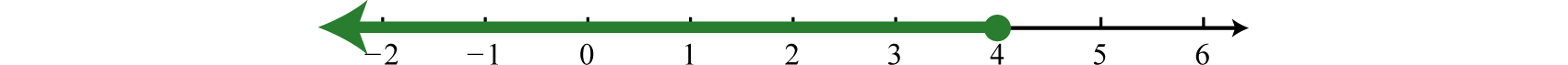Figure 1.8.4

Interval notation: $$(−∞, 4]$$

Exercise $$\PageIndex{1}$$

Solve and graph the solution set: $$10 - 5 ( 2 x + 3 ) \leq 25$$

$$[ - 3 , \infty )$$;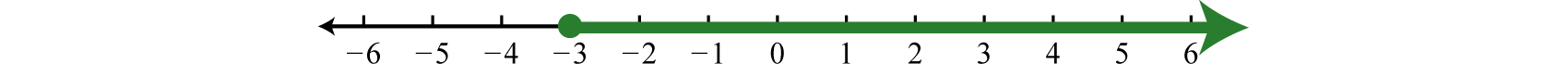Figure 1.8.5

Compound Inequalities

Following are some examples of compound linear inequalities:

 $$- 13 < 3 x - 7 < 17$$ $$4 x + 5 \leq - 15 \text { or } 6 x - 11 > 7$$

Table 1.8.5

These compound inequalities142 are actually two inequalities in one statement joined by the word and or by the word or. For example,

$$- 13 < 3 x - 7 < 17$$

is a compound inequality because it can be decomposed as follows:

$$- 13 < 3 x - 7 \text { and } 3 x - 7 < 17$$

We can solve each inequality individually; the intersection of the two solution sets solves the original compound inequality. While this method works, there is another method that usually requires fewer steps. Apply the properties of this section to all three parts of the compound inequality with the goal of isolating the variable in the middle of the statement to determine the bounds of the solution set.

Example $$\PageIndex{6}$$:

Solve and graph the solution set: $$−13<3x−7<17$$.

Solution:

$$\begin{array} { c } { - 13 < 3 x - 7 < 17 } \\ { - 13 \color{Cerulean}{+ 7}\color{Black}{ <} 3 x - 7 \color{Cerulean}{+ 7}\color{Black}{ <} 17 \color{Cerulean}{+ 7} } \\ { - 6 < 3 x < 24 } \\ { \frac { - 6 } { \color{Cerulean}{3} } \color{Black}{<} \frac { 3 x } { \color{Cerulean}{3} } \color{Black}{<} \frac { 24 } { \color{Cerulean}{3} } } \\ { - 2 < x < 8 } \end{array}$$Figure 1.8.6

Interval notation: $$(−2,8)$$

Example $$\PageIndex{7}$$:

Solve and graph the solution set: $$\frac{5}{6}≤\frac{1}{3}(\frac{1}{2}x+4)<2$$.

Solution:

$$\begin{array} { c } { \frac { 5 } { 6 } \leq \frac { 1 } { 3 } \left( \frac { 1 } { 2 } x + 4 \right) < 2 } \\ { \frac { 5 } { 6 } \leq \frac { 1 } { 6 } x + \frac { 4 } { 3 } < 2 } \\ { \color{Cerulean}{6}\color{Black}{ \cdot} \left( \frac { 5 } { 6 } \right) \leq \color{Cerulean}{6}\color{Black}{ \cdot} \left( \frac { 1 } { 6 } x + \frac { 4 } { 3 } \right) < \color{Cerulean}{6}\color{Black}{ \cdot} ( 2 ) } \\ { 5 \leq x + 8 < 12 } \\ { 5 \color{Cerulean}{- 8}\color{Black}{ \leq} x + 8 \color{Cerulean}{- 8}\color{Black}{ <} 12 \color{Cerulean}{- 8} } \\ { - 3 \leq x < 4 } \end{array}$$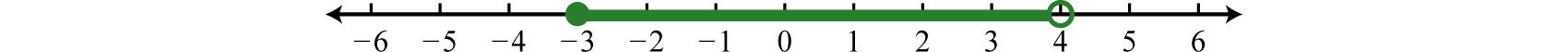Figure 1.8.7

Interval notation $$[−3,4)$$

It is important to note that when multiplying or dividing all three parts of a compound inequality by a negative number, you must reverse all of the inequalities in the statement. For example:

$$\begin{array} { l } { - 10 < - 2 x < 20 } \\ { \frac { - 10 } { \color{Cerulean}{- 2} } \color{OliveGreen}{>} \frac { \color{Black}{- 2 x} } { \color{Cerulean}{ - 2} } \color{OliveGreen}{>} \frac { \color{Black}{20} } { \color{Cerulean}{- 2} } } \\ { 5 > x > - 10 } \end{array}$$

The answer above can be written in an equivalent form, where smaller numbers lie to the left and the larger numbers lie to the right, as they appear on a number line.

$$- 10 < x < 5$$

Use interval notation, write: $$(-10, 5)$$.

Exercise $$\PageIndex{2}$$

Solve and graph the solution set: $$−3≤−3(2x−3)<15$$.

$$(-1,2]$$;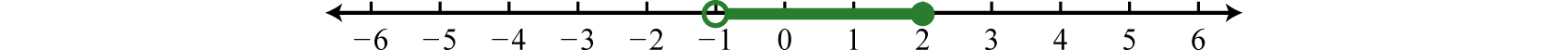Figure 1.8.8

For compound inequalities with the word “or” you work both inequalities separately and then consider the union of the solution sets. Values in this union solve either inequality.

Example $$\PageIndex{8}$$:

Solve and graph the solution set: $$4x+5≤−15$$  or  $$6x−11>7$$.

Solution:

Solve each inequality and form the union by combining the solution sets.

 \begin{aligned} 4 x + 5 & \leq - 15 \\ 4 x & \leq - 20 \\ x & \leq - 5 \end{aligned} or $$\begin{array} { r } { 6 x - 11 > 7 } \\ { 6 x > 18 } \\ { x > 3 } \end{array}$$

Table 1.8.6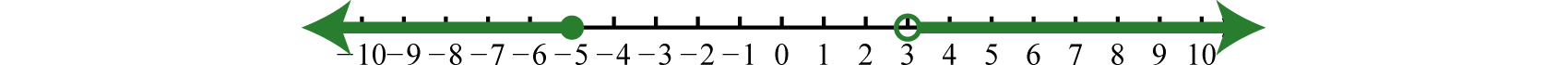Figure 1.8.9

Interval notation $$(−∞,−5]∪(3,∞)$$

Exercise $$\PageIndex{3}$$

Solve and graph the solution set: $$5 ( x - 3 ) < - 20 \text { or } 2 ( 5 - 3 x ) < 1$$.

$$( - \infty , - 1 ) \cup \left( \frac { 3 } { 2 } , \infty \right)$$Figure 1.8.10

Applications of Linear Inequalities

Some of the key words and phrases that indicate inequalities are summarized below:

Key Phrases Translation
A number is at least $$5$$. $$x\geq 5$$
A number is $$5$$ or more inclusive. $$x\geq 5$$
A number is at most $$3$$. $$x\leq 3$$
A number is $$3$$ or less inclusive. $$x\leq 3$$
A number is strictly less than $$4$$. $$x<4$$
A number is less than $$4$$, noninclusive. $$x<4$$
A number is greater than $$7$$. $$x>7$$
A number is more than $$7$$, noninclusive. $$x>7$$
A number is in between $$2$$ and $$10$$. $$2<x<10$$
A number is at least $$5$$ and at most $$15$$. $$5\leq x\leq 15$$
A number may range from $$5$$ to $$15$$. $$5\leq x\leq 15$$

Table 1.8.7

As with all applications, carefully read the problem several times and look for key words and phrases. Identify the unknowns and assign variables. Next, translate the wording into a mathematical inequality. Finally, use the properties you have learned to solve the inequality and express the solution graphically or in interval notation.

Example $$\PageIndex{9}$$:

Seven less than $$3$$ times the sum of a number and $$5$$ is at most $$11$$. Find all numbers that satisfy this condition.

Solution:

First, choose a variable for the unknown number and identify the key words and phrases.

Let n represent the unknown indicated by “a number.”Figure 1.8.11

Solve for n.

\begin{aligned} 3 ( n + 5 ) - 7 & \leq 11 \\ 3 n + 15 - 7 & \leq 11 \\ 3 n + 8 & \leq 11 \\ 3 n & \leq 3 \\ n & \leq 1 \end{aligned}

Any number less than or equal to $$1$$ will satisfy the statement.

Example $$\PageIndex{10}$$:

To earn a B in a mathematics course the test average must be at least $$80$$% and less than $$90$$%. If a student earned $$92$$%, $$96$$%, $$79$$%, and $$83$$% on the first four tests, what must she score on the fifth test to earn a B?

Solution:

Set up a compound inequality where the test average is between $$80$$% and $$90$$%. In this case, include the lower bound, $$80$$.

Let x represent the score on the fifth test.

\begin{aligned} 80\quad \leq\quad & \color{Cerulean}{ test\: average }\quad\quad\quad\quad < 90 \\ 80\quad \leq \quad & \frac { 92 + 96 + 79 + 83 + x } { 5 }<90 \\ \color{Cerulean}{5}\color{Black}{ \cdot} 80 \leq\quad & \color{Cerulean}{5}\color{Black}{ \cdot} \frac { 350 + x } { 5 } \quad\quad\quad\quad\quad<\color{Cerulean}{5}\color{Black}{\cdot} 90 \\ 400 \leq\quad & 350 + x \quad\quad\quad\quad\quad\quad\:\:\:<45 \\ 50 \leq\quad & x\quad\quad\quad\quad\quad\quad\quad\quad\quad\:\:<100 \end{aligned}

She must earn a score of at least $$50$$% and less than $$100$$%.

In the previous example, the upper bound $$100$$% was not part of the solution set. What would happen if she did earn a $$100$$% on the fifth test?

\begin{aligned} \text {average} & = \frac { 92 + 96 + 79 + 83 + \color{Cerulean}{100} } {\color{Black}{ 5} } \\ & = \frac { 450 } { 5 } \\ & = 90 \end{aligned}

As we can see, her average would be $$90$$%, which would earn her an A.

Key Takeaways

• Inequalities typically have infinitely many solutions. The solutions are presented graphically on a number line or using interval notation or both.
• All but one of the rules for solving linear inequalities are the same as solving linear equations. If you divide or multiply an inequality by a negative number, reverse the inequality to obtain an equivalent inequality.
• Compound inequalities involving the word “or” require us to solve each inequality and form the union of each solution set. These are the values that solve at least one of the given inequalities.
• Compound inequalities involving the word “and” require the intersection of the solution sets for each inequality. These are the values that solve both or all of the given inequalities.
• The general guidelines for solving word problems apply to applications involving inequalities. Be aware of a new list of key words and phrases that indicate a mathematical setup involving inequalities.

Exercise $$\PageIndex{4}$$

Determine whether or not the given value is a solution.

1. $$5 x - 1 < - 2 ; x = - 1$$
2. $$- 3 x + 1 > - 10 ; x = 1$$
3. $$2 x - 3 < - 5 ; x = 1$$
4. $$5 x - 7 < 0 ; x = 2$$
5. $$9 y - 4 \geq 5 ; y = 1$$
6. $$- 6 y + 1 \leq 3 ; y = - 1$$
7. $$12 a + 3 \leq - 2 ; a = - \frac { 1 } { 3 }$$
8. $$25 a - 2 \leq - 22 ; a = - \frac { 4 } { 5 }$$
9. $$- 10 < 2 x - 5 < - 5 ; x = - \frac { 1 } { 2 }$$
10. $$3 x + 8 < - 2 \text { or } 4 x - 2 > 5 ; x = 2$$

1. Yes

3. No

5. Yes

7. No

9. Yes

Exercise $$\PageIndex{5}$$

Graph all solutions on a number line and provide the corresponding interval notation.

1. $$3 x + 5 > - 4$$
2. $$2 x + 1 > - 1$$
3. $$5 - 6 y < - 1$$
4. $$7 - 9 y > 43$$
5. $$6 - a \leq 6$$
6. $$- 2 a + 5 > 5$$
7. $$\frac { 5 x + 6 } { 3 } \leq 7$$
8. $$\frac { 4 x + 11 } { 6 } \leq \frac { 1 } { 2 }$$
9. $$\frac { 1 } { 2 } y + \frac { 5 } { 4 } \geq \frac { 1 } { 4 }$$
10. $$\frac { 1 } { 12 } y + \frac { 2 } { 3 } \leq \frac { 5 } { 6 }$$
11. $$2 ( 3 x + 14 ) < - 2$$
12. $$5 ( 2 y + 9 ) > - 15$$
13. $$5 - 2 ( 4 + 3 y ) \leq 45$$
14. $$- 12 + 5 ( 5 - 2 x ) < 83$$
15. $$6 ( 7 - 2 a ) + 6 a \leq 12$$
16. $$2 a + 10 ( 4 - a ) \geq 8$$
17. $$9 ( 2 t - 3 ) - 3 ( 3 t + 2 ) < 30$$
18. $$- 3 ( t - 3 ) - ( 4 - t ) > 1$$
19. $$\frac { 1 } { 2 } ( 5 x + 4 ) + \frac { 5 } { 6 } x > - \frac { 4 } { 3 }$$
20. $$\frac { 2 } { 5 } + \frac { 1 } { 6 } ( 2 x - 3 ) \geq \frac { 1 } { 15 }$$
21. $$5 x - 2 ( x - 3 ) < 3 ( 2 x - 1 )$$
22. $$3 ( 2 x - 1 ) - 10 > 4 ( 3 x - 2 ) - 5 x$$
23. $$- 3 y \geq 3 ( y + 8 ) + 6 ( y - 1 )$$
24. $$12 \leq 4 ( y - 1 ) + 2 ( 2 y + 1 )$$
25. $$- 2 ( 5 t - 3 ) - 4 > 5 ( - 2 t + 3 )$$
26. $$- 7 ( 3 t - 4 ) > 2 ( 3 - 10 t ) - t$$
27. $$\frac { 1 } { 2 } ( x + 5 ) - \frac { 1 } { 3 } ( 2 x + 3 ) > \frac { 7 } { 6 } x + \frac { 3 } { 2 }$$
28. $$- \frac { 1 } { 3 } ( 2 x - 3 ) + \frac { 1 } { 4 } ( x - 6 ) \geq \frac { 1 } { 12 } x - \frac { 5 } { 4 }$$
29. $$4 ( 3 x + 4 ) \geq 3 ( 6 x + 5 ) - 6 x$$
30. $$1 - 4 ( 3 x + 7 ) < - 3 ( x + 9 ) - 9 x$$
31. $$6 - 3 ( 2 a - 1 ) \leq 4 ( 3 - a ) + 1$$
32. $$12 - 5 ( 2 a + 6 ) \geq 2 ( 5 - 4 a ) - a$$

1. $$( - 3 , \infty )$$;Figure 1.8.12

3. $$( 1 , \infty )$$;Figure 1.8.13

5. $$[ 0 , \infty )$$;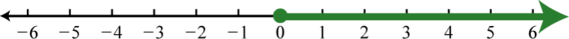Figure 1.8.14

7. $$( - \infty , 3 ]$$;Figure 1.8.15

9. $$[ - 2 , \infty )$$;Figure 1.8.16

11. $$( - \infty , - 5 )$$;Figure 1.8.17

13. $$[ - 8 , \infty )$$;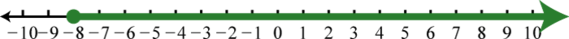Figure 1.8.18

15. $$[ 5 , \infty )$$;Figure 1.8.19

17. $$( - \infty , 7 )$$;Figure 1.8.20

19. $$( - 1 , \infty )$$;Figure 1.8.21

21. $$( 3 , \infty )$$;Figure 1.8.22

23. $$\left( - \infty , - \frac { 3 } { 2 } \right]$$;Figure 1.8.23

25. $$\emptyset$$;Figure 1.8.24

27. $$( - \infty , 0 )$$;Figure 1.8.25

29. $$\mathbb { R }$$;Figure 1.8.26

31. $$[ - 2 , \infty )$$;Figure 1.8.27

Exercise $$\PageIndex{6}$$

Graph all solutions on a number line and provide the corresponding interval notation.

1. $$- 1 < 2 x + 1 < 9$$
2. $$- 4 < 5 x + 11 < 16$$
3. $$- 7 \leq 6 y - 7 \leq 17$$
4. $$- 7 \leq 3 y + 5 \leq 2$$
5. $$- 7 < \frac { 3 x + 1 } { 2 } \leq 8$$
6. $$- 1 \leq \frac { 2 x + 7 } { 3 } < 1$$
7. $$- 4 \leq 11 - 5 t < 31$$
8. $$15 < 12 - t \leq 16$$
9. $$- \frac { 1 } { 3 } \leq \frac { 1 } { 6 } a + \frac { 1 } { 3 } \leq \frac { 1 } { 2 }$$
10. $$- \frac { 1 } { 6 } < \frac { 1 } { 3 } a + \frac { 5 } { 6 } < \frac { 3 } { 2 }$$
11. $$5 x + 2 < - 3 \text { or } 7 x - 6 > 15$$
12. $$4 x + 15 \leq - 1 \text { or } 3 x - 8 \geq - 11$$
13. $$8 x - 3 \leq 1 \text { or } 6 x - 7 \geq 8$$
14. $$6 x + 1 < - 3 \text { or } 9 x - 20 > - 5$$
15. $$8 x - 7 < 1 \text { or } 4 x + 11 > 3$$
16. $$10 x - 21 < 9 \text { or } 7 x + 9 \geq 30$$
17. $$7 + 2 y < 5 \text { or } 20 - 3 y > 5$$
18. $$5 - y < 5 \text { or } 7 - 8 y \leq 23$$
19. $$15 + 2 x < - 15 \text { or } 10 - 3 x > 40$$
20. $$10 - \frac { 1 } { 3 } x \leq 5 \text { or } 5 - \frac { 1 } { 2 } x \leq 15$$
21. $$9 - 2 x \leq 15 \text { and } 5 x - 3 \leq 7$$
22. $$5 - 4 x > 1 \text { and } 15 + 2 x \geq 5$$
23. $$7 y - 18 < 17 \text { and } 2 y - 15 < 25$$
24. $$13 y + 20 \geq 7 \text { and } 8 + 15 y > 8$$
25. $$5 - 4 x \leq 9 \text { and } 3 x + 13 \leq 1$$
26. $$17 - 5 x \geq 7 \text { and } 4 x - 7 > 1$$
27. $$9 y + 20 \leq 2 \text { and } 7 y + 15 \geq 1$$
28. $$21 - 6 y \leq 3 \text { and } - 7 + 2 y \leq - 1$$
29. $$- 21 < 6 ( x - 3 ) < - 9$$
30. $$0 \leq 2 ( 2 x + 5 ) < 8$$
31. $$- 15 \leq 5 + 4 ( 2 y - 3 ) < 17$$
32. $$5 < 8 - 3 ( 3 - 2 y ) \leq 29$$
33. $$5 < 5 - 3 ( 4 + t ) < 17$$
34. $$- 3 \leq 3 - 2 ( 5 + 2 t ) \leq 21$$
35. $$- 40 < 2 ( x + 5 ) - ( 5 - x ) \leq - 10$$
36. $$- 60 \leq 5 ( x - 4 ) - 2 ( x + 5 ) \leq 15$$
37. $$- \frac { 1 } { 2 } < \frac { 1 } { 30 } ( x - 10 ) < \frac { 1 } { 3 }$$
38. $$- \frac { 1 } { 5 } \leq \frac { 1 } { 15 } ( x - 7 ) \leq \frac { 1 } { 3 }$$
39. $$- 1 \leq \frac { a + 2 ( a - 2 ) } { 5 } \leq 0$$
40. $$0 < \frac { 5 + 2 ( a - 1 ) } { 6 } < 2$$

1. $$(- 1,4 )$$;Figure 1.8.28

3. $$[0,4]$$;Figure 1.8.29

5. $$(−5,5]$$;Figure 1.8.30

7. $$(−4,3]$$;Figure 1.8.31

9. $$[−4,1]$$;Figure 1.8.32

11. $$(−∞,−1)∪(3,∞)$$;Figure 1.8.33

13. $$(−∞,12]∪[52,∞)$$;Figure 1.8.34

15. $$ℝ$$;Figure 1.8.35

17. $$(−∞,5)$$;Figure 1.8.36

19. $$(−∞,−10)$$;Figure 1.8.37

21. $$[−3,2]$$;Figure 1.8.38

23. $$(−∞,5)$$;Figure 1.8.39

25. $$Ø$$;Figure 1.8.40

27. $$−2$$;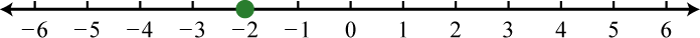Figure 1.8.41

29. $$(−12,32)$$;Figure 1.8.42

31. $$[−1,3)$$;Figure 1.8.43

33. $$(−8,−4)$$;Figure 1.8.44

35. $$(−15,−5]$$;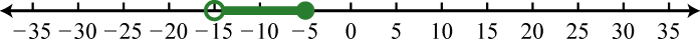Figure 1.8.45

37. $$(−5,20)$$;Figure 1.8.46

39. $$[−13, 43]$$;Figure 1.8.47

Exercise $$\PageIndex{7}$$

Find all numbers that satisfy the given condition.

1. Three less than twice the sum of a number and $$6$$ is at most $$13$$.
2. Five less than $$3$$ times the sum of a number and $$4$$ is at most $$10$$.
3. Five times the sum of a number and $$3$$ is at least $$5$$.
4. Three times the difference between a number and $$2$$ is at least $$12$$.
5. The sum of $$3$$ times a number and $$8$$ is between $$2$$ and $$20$$.
6. Eight less than twice a number is between $$−20$$ and $$−8$$.
7. Four subtracted from three times some number is between $$−4$$ and $$14$$.
8. Nine subtracted from $$5$$ times some number is between $$1$$ and $$11$$.

1. $$( - \infty , 2 ]$$

3. $$[ - 2 , \infty )$$

5. $$( - 2,4 )$$

7. $$( 0,6 )$$

Exercise $$\PageIndex{8}$$

Set up an algebraic inequality and then solve.

1. With a golf club membership, costing $$120$$ per month, each round of golf costs only $$35.00$$. How many rounds of golf can a member play if he wishes to keep his costs $$270$$ per month at most?
2. A rental truck costs $$95$$ per day plus $$0.65$$ per mile driven. How many miles can be driven on a one-day rental to keep the cost at most $$120$$?
3. Mark earned $$6, 7$$, and $$10$$ points out of $$10$$ on the first three quizzes. What must he score on the fourth quiz to average at least $$8$$?
4. Joe earned scores of $$78, 82, 88$$ and $$70$$ on his first four algebra exams. What must he score on the fifth exam to average at least $$80$$?
5. A gymnast scored $$13.2, 13.0, 14.3, 13.8$$, and $$14.6$$ on the first five events. What must he score on the sixth event to average at least $$14.0$$?
6. A dancer scored $$7.5$$ and $$8.2$$ from the first two judges. What must her score from the third judge come in as if she is to average $$8.4$$ or higher?
7. If two times an angle is between $$180$$ degrees and $$270$$ degrees, then what are the bounds of the original angle?
8. The perimeter of a square must be between $$120$$ inches and $$460$$ inches. Find the length of all possible sides that satisfy this condition.
9. A computer is set to shut down if the temperature exceeds $$45$$°C. Give an equivalent statement using degrees Fahrenheit. Hint: $$C = \frac{5}{9} (F − 32)$$.
10. A certain antifreeze is effective for a temperature range of $$−35$$°C to $$120$$°C. Find the equivalent range in degrees Fahrenheit.

1. Members may play $$4$$ rounds or fewer.

3. Mark must earn at least $$9$$ points on the fourth quiz.

5. He must score a $$15.1$$ on the sixth event.

7. The angle is between $$90$$ degrees and $$135$$ degrees.

9. The computer will shut down when the temperature exceeds $$113$$°F.

Exercise $$\PageIndex{9}$$

1. Often students reverse the inequality when solving $$5x + 2 < −18$$? Why do you think this is a common error? Explain to a beginning algebra student why we do not.
2. Conduct a web search for “solving linear inequalities.” Share a link to website or video tutorial that you think is helpful.
3. Write your own $$5$$ key takeaways for this entire chapter. What did you find to be review and what did you find to be new? Share your thoughts on the discussion board.

138Linear expressions related with the symbols $$≤, <, ≥,$$ and $$>$$ .